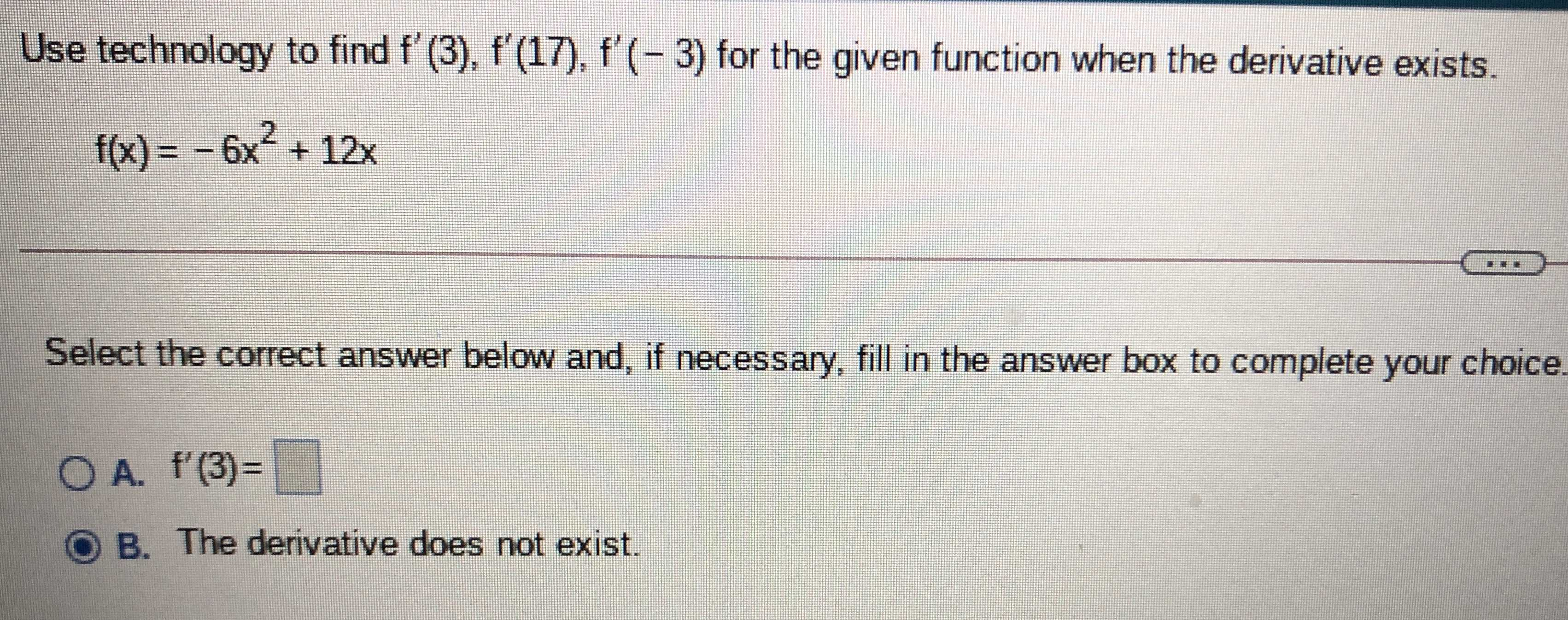### Still have math questions?

Algebra
QuestionUse technology to find $$f ^ { \prime } ( 3 ) , f ^ { \prime } ( 17 ) , f ^ { \prime } ( - 3 )$$ for the given function when the derivative exists.

$$f ( x ) = - 6 x ^ { 2 } + 12 x$$

Select the correct answer below and, if necessary, fill in the answer box to complete your choice.

A. $$f ^ { \prime } ( 3 ) = \square$$

B. The derivative does not exist.

$$f'(3) = −24$$